# Suppose f is a continuous on R and f(x + y) = f(x) + f(y) for...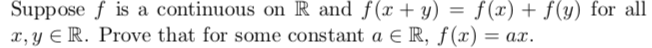Suppose f is a continuous on R and f(x + y) = f(x) + f(y) for all X,Y E R. Prove that for some constant a ER, f(x) = ar.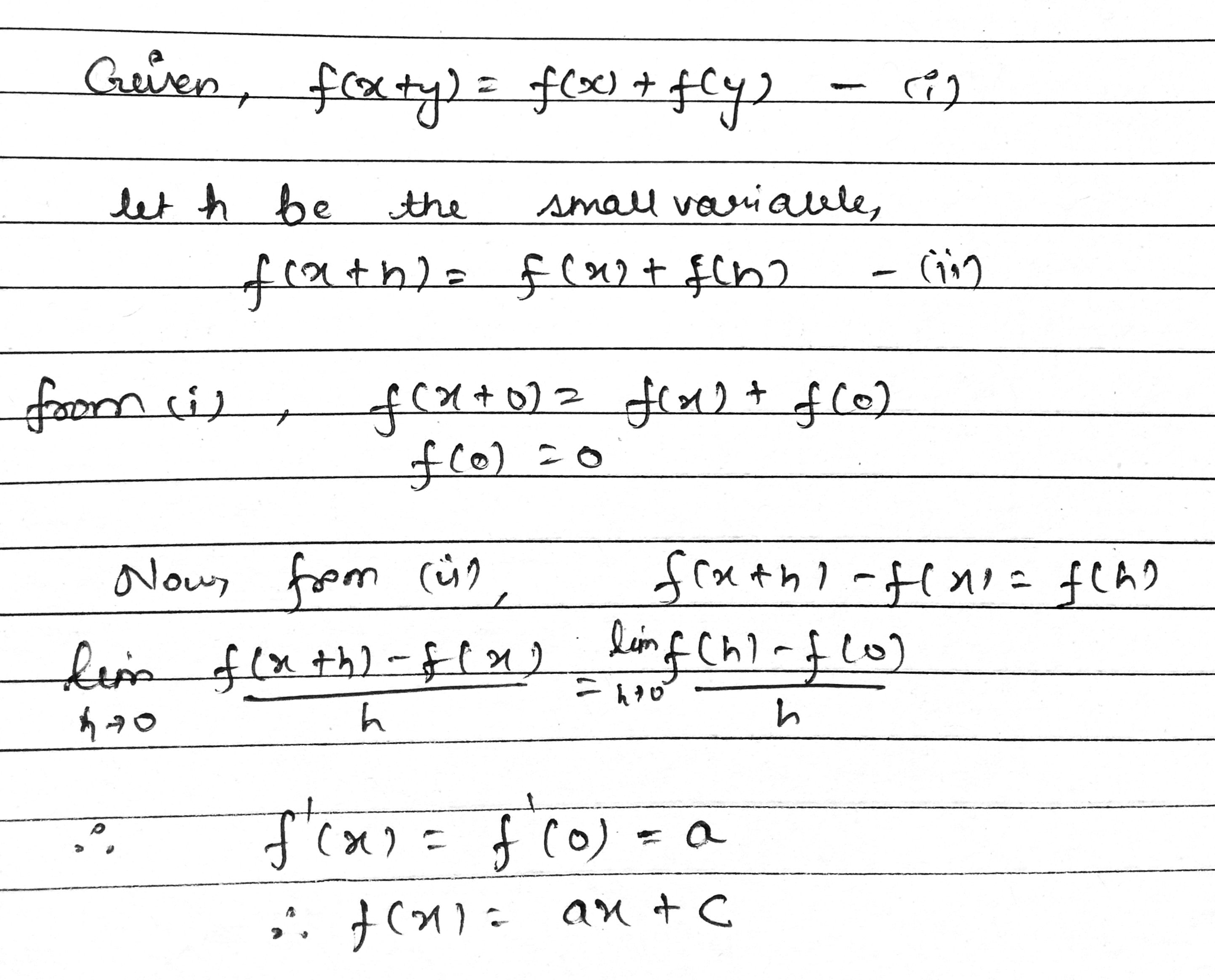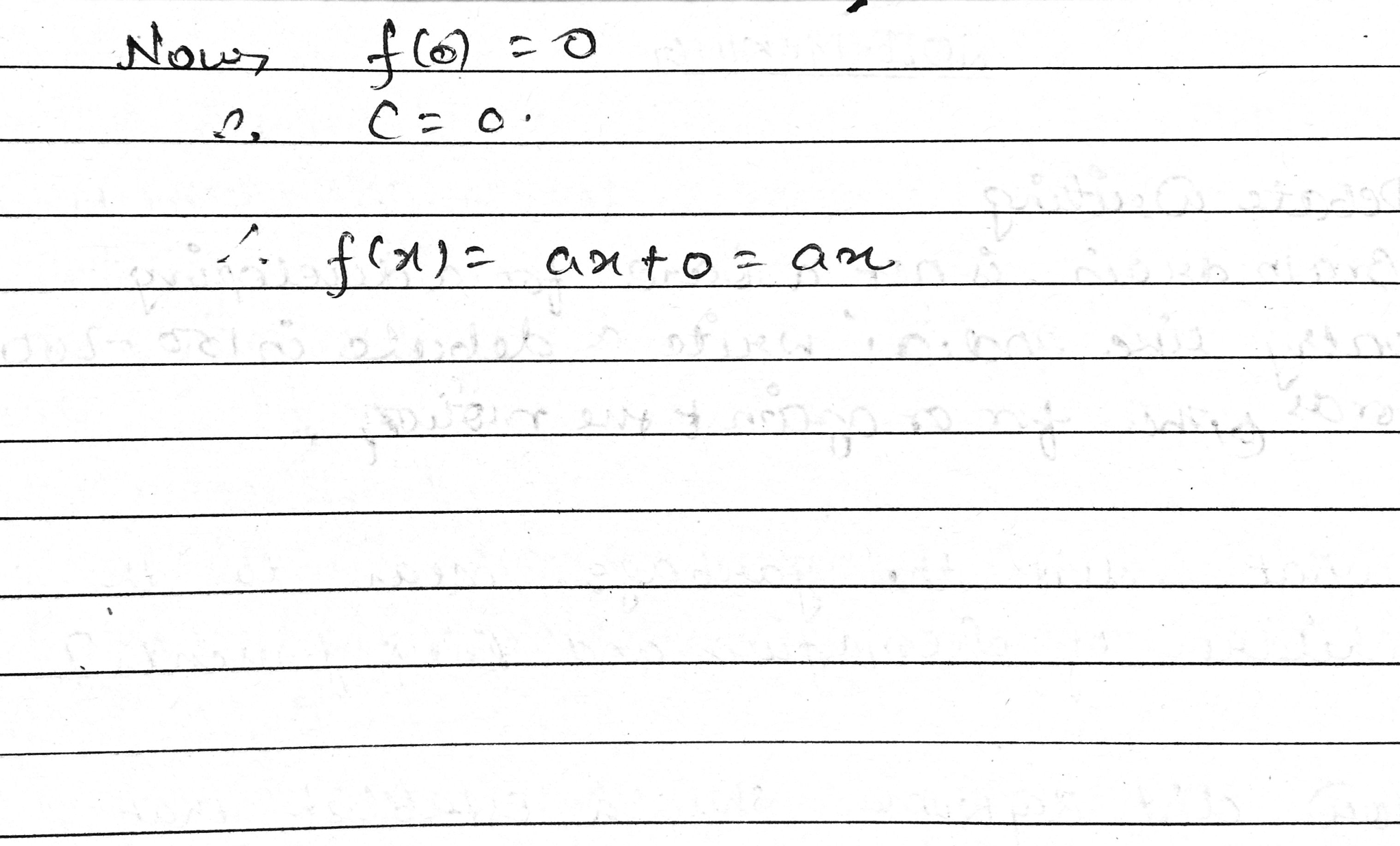##### Add Answer of: Suppose f is a continuous on R and f(x + y) = f(x) + f(y) for...
Similar Homework Help Questions
• ### Suppose f is a continuous on R and f(x + y) = f(x) + f(y) for...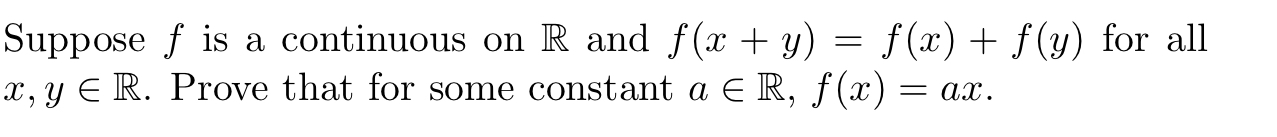Suppose f is a continuous on R and f(x + y) = f(x) + f(y) for all x, y ∈ R. Prove that for some constant a ∈ R, f(x) = ax. Suppose f is a continuous on R and f(x + y) = f(x) + f(y) for all X, Y E R. Prove that for some constant a ER, f(x) = ax.

• ### ULUM turu Problem 1. Assume that f:R R is continuous and satisfies f(x) - f(y) =...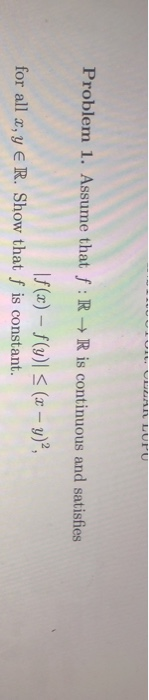ULUM turu Problem 1. Assume that f:R R is continuous and satisfies f(x) - f(y) = (x - y)?, for all x, y ER. Show that f is constant.

• ### S f(r)da= g(x)dz. Prove a,bsuch that (8) Suppose f and g are continuous functions on that...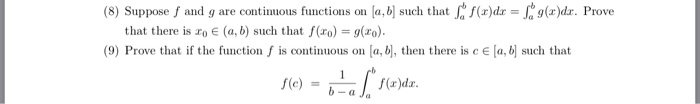S f(r)da= g(x)dz. Prove a,bsuch that (8) Suppose f and g are continuous functions on that there is ro e (a, b) such that f(ro) = g(xo). (9) Prove that if the function f is continuous on a, b, then there is c E [a, b such that f(x)dax a Ja f(e) S f(r)da= g(x)dz. Prove a,bsuch that (8) Suppose f and g are continuous functions on that there is ro e (a, b) such that f(ro) = g(xo). (9)...

• ### (1) If f: R₃ R a continuous function such that f(x)² > 0 for all xER....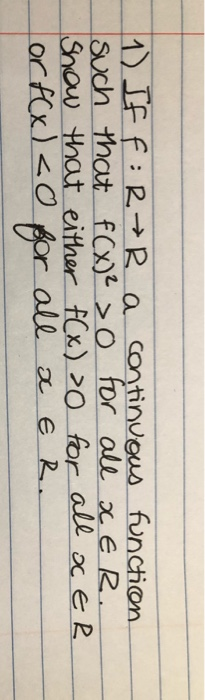(1) If f: R₃ R a continuous function such that f(x)² > 0 for all xER. Show that either f(x) >0 for all a ER or f(x) <0 all X E R.

• ### Real analysis 2. Consider the following three definitions: A function f : R-+R is lax-continuous at a E R provided for...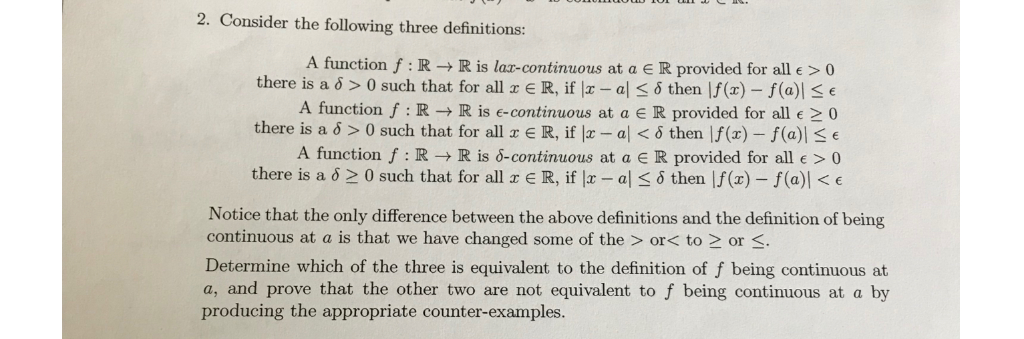Real analysis 2. Consider the following three definitions: A function f : R-+R is lax-continuous at a E R provided for all e > 0 there is a 6 > 0 such that for all r E R, if x - al6 then |f(x)- f (a)e A function f : R - R is e-continuous at a E R provided for all e >0 there is a 6 > 0 such that for all r E R, if |a- a...

• ### Suppose that k e N and that f R"-R is homogeneous of order k: that is, that 'f(px)- kf(x) for all x є Rn and all є R. If f is differentiable on R", prove that af Экп af axi (Xi , . . . ,...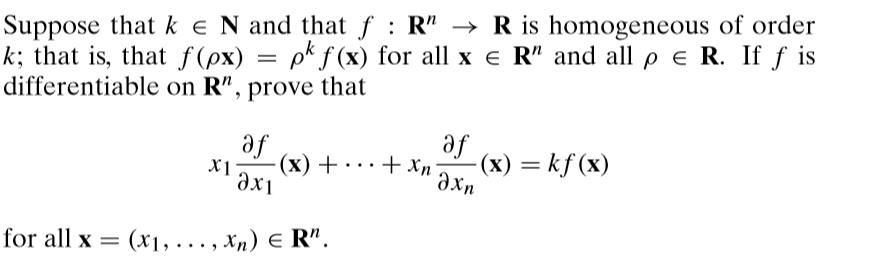Suppose that k e N and that f R"-R is homogeneous of order k: that is, that 'f(px)- kf(x) for all x є Rn and all є R. If f is differentiable on R", prove that af Экп af axi (Xi , . . . , xn) ER". for all x Suppose that k e N and that f R"-R is homogeneous of order k: that is, that 'f(px)- kf(x) for all x є Rn and all є R. If...

• ### 3. Suppose f : [0,) + R is a continuous function and that L limf(x) exists...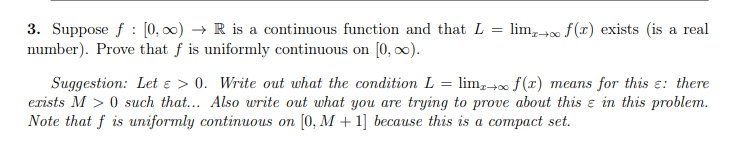3. Suppose f : [0,) + R is a continuous function and that L limf(x) exists is a real number). Prove that f is uniformly continuous on (0,.). Suggestion: Let e > 0. Write out what the condition L = lim,+ f(t) means for this e: there erists M > 0 such that... Also write out what you are trying to prove about this e in this problem. Note that f is uniformly continuous on (0.M +1] because this is...

• ### Suppose R is the shaded region in the figure, and f(x, y) is a continuous function on R. Find the limits of integration for the following iterated integrals. 3- (a) f(x, y) dA f(x, g) dy dz B- | | f...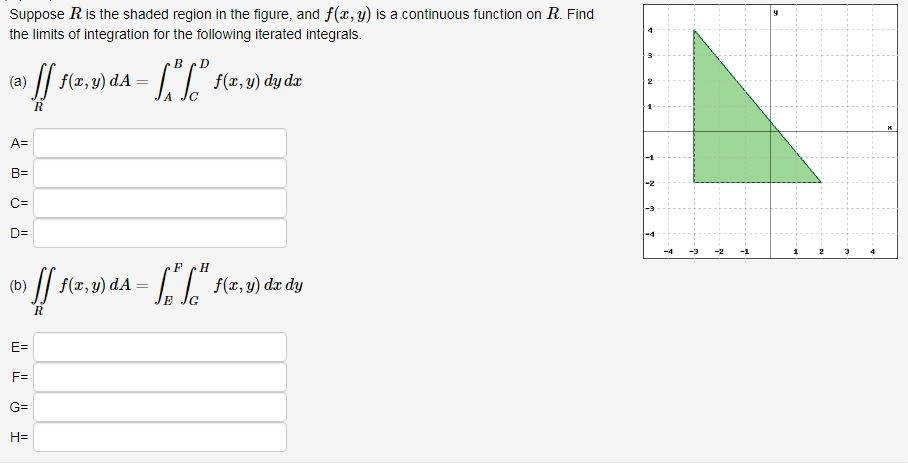Suppose R is the shaded region in the figure, and f(x, y) is a continuous function on R. Find the limits of integration for the following iterated integrals. 3- (a) f(x, y) dA f(x, g) dy dz B- | | f(x,v) dA= f(z, g) dx dy (b) E- F: We were unable to transcribe this image Suppose R is the shaded region in the figure, and f(x, y) is a continuous function on R. Find the limits of integration for...

• ### Problem 2 Suppose two continuous random variables (X, Y) ~ f(x,y). (1) Prove E(X +Y) =...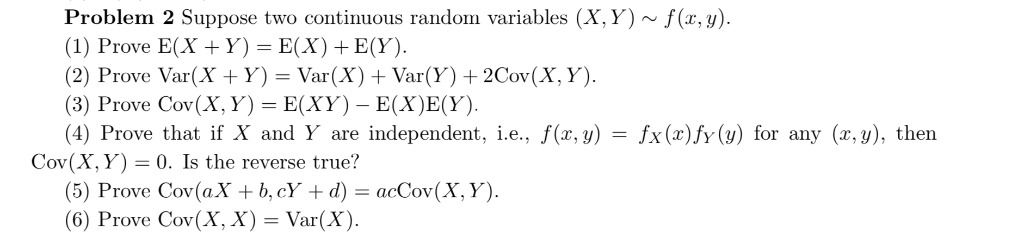Problem 2 Suppose two continuous random variables (X, Y) ~ f(x,y). (1) Prove E(X +Y) = E(X)+ E(Y). (2) Prove Var(X + Y) = Var(X) + Var(Y)2Cov(X, Y). (3) Prove Cov(X, Y) E(XY)- E(X)E(Y). (4) Prove that if X and Y are independent, i.e., f(x, y) Cov(X, Y) 0. Is the reverse true? (5) Prove Cov (aX b,cY + d) = acCov(X, Y). (6) Prove Cov(X, X) = Var(X) fx (x)fy(y) for any (x,y), then =

• ### Can you help with this? Thank you always. Suppose that the function f : R-+ R is continuous at the point xo and that f(...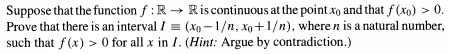Can you help with this? Thank you always. Suppose that the function f : R-+ R is continuous at the point xo and that f(xo) > 0. Prove that there is an interval 1 (x,-1/n, xo + 1 /n), where n is a natural number, such that f (x) >0 for all x in I. (Hint: Argue by contradiction.) Suppose that the function f : R-+ R is continuous at the point xo and that f(xo) > 0. Prove that...

Free Homework App